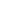The next term in the following sequence 2, 9, 28, 65, ____

a) 132
b) 126
c) 129
d) 125
KPSC Divisional Accountant-15

 126. Solution 2 = (1*1*1) + 1 9 = (2*2*2) + 1 28 = (3*3*3) + 1 65 = (4*4*4) + 1 126 = (5*5*5) + 1
Share :
+
Next
« Prev
Prev
Next »